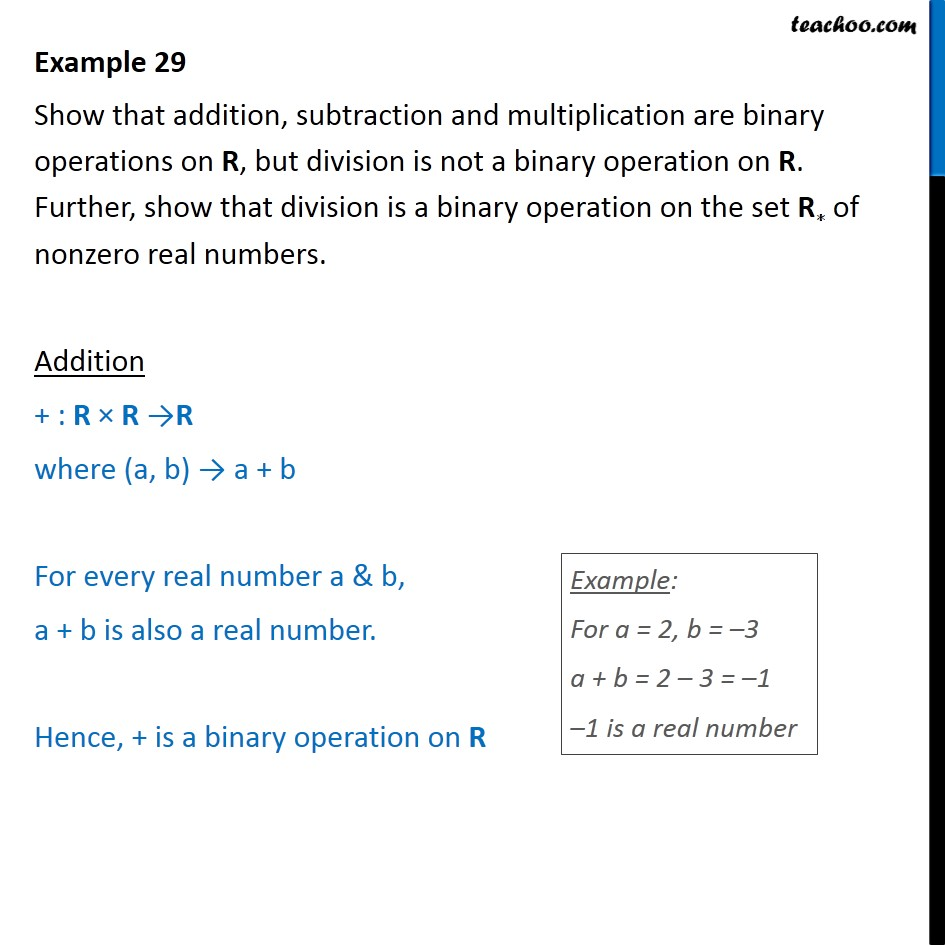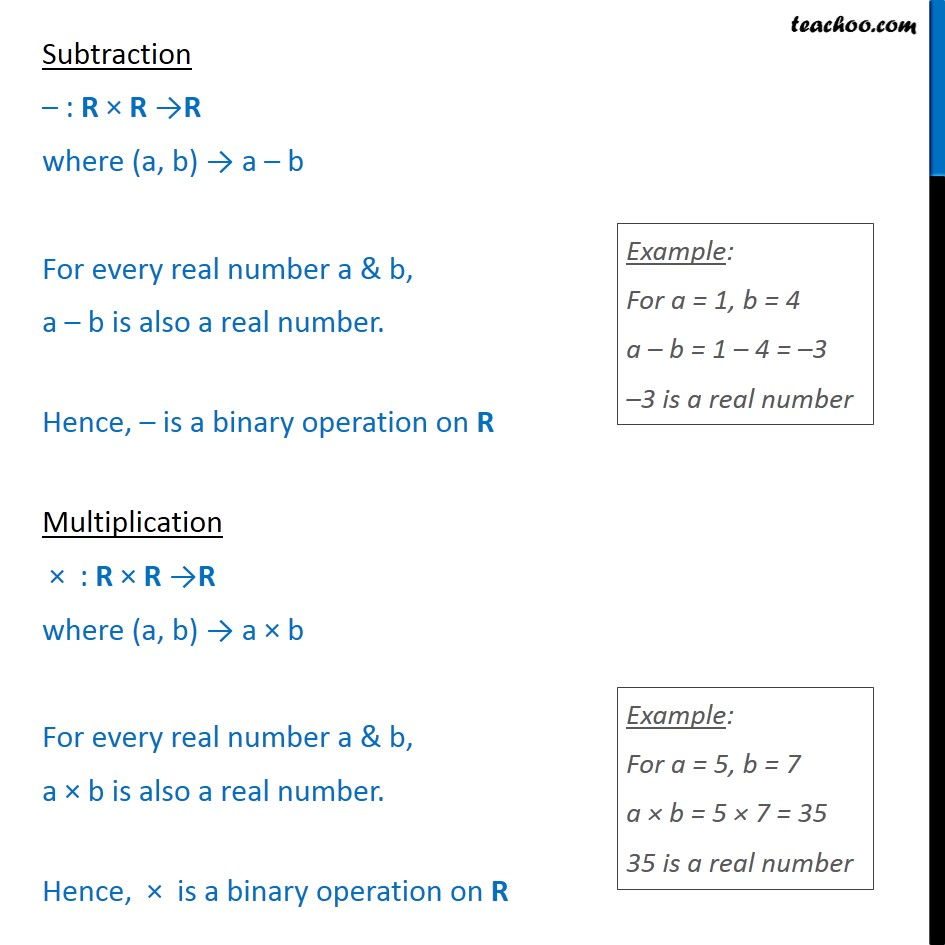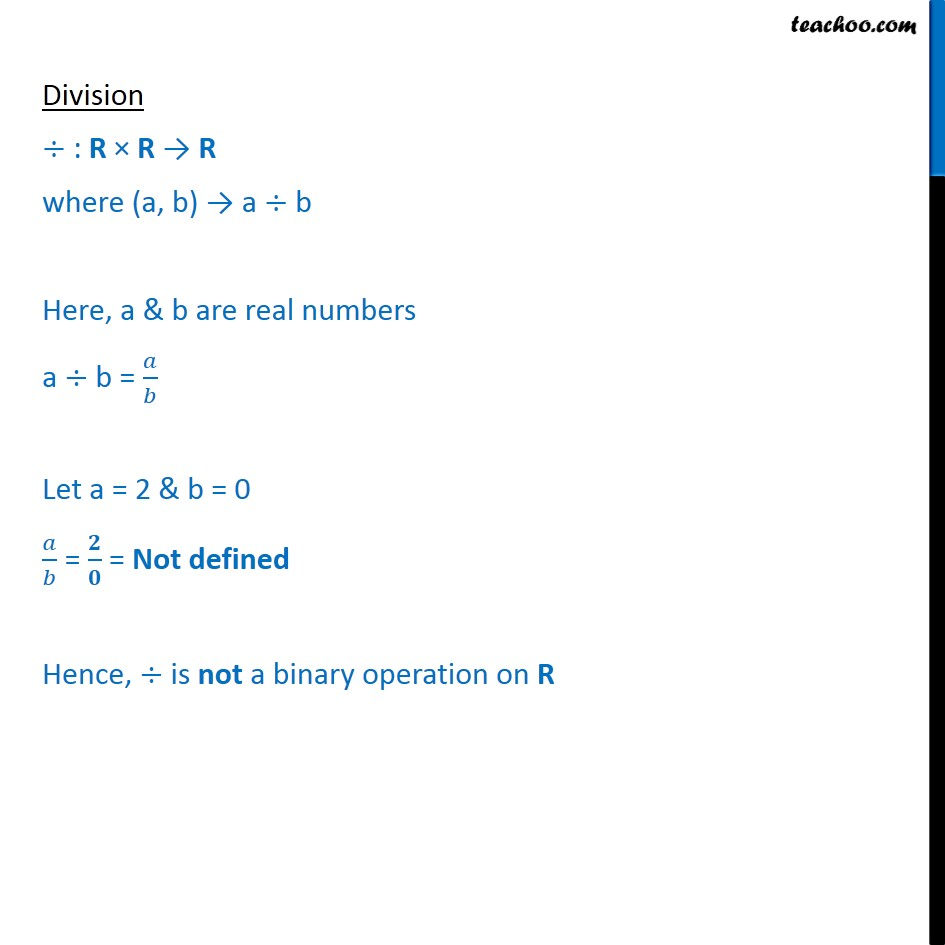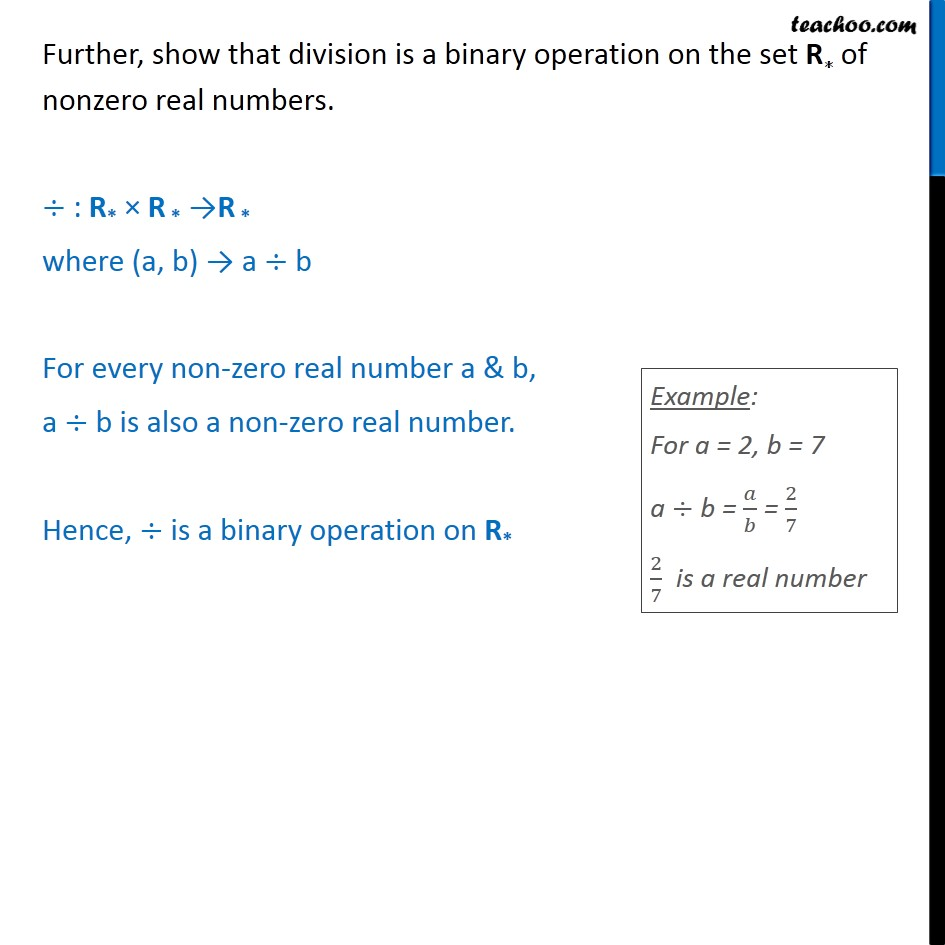Examples

Chapter 1 Class 12 Relation and Functions (Term 1)
Serial order wiseNow learn Economics at Teachoo for Class 12

### Transcript

Example 29 Show that addition, subtraction and multiplication are binary operations on R, but division is not a binary operation on R. Further, show that division is a binary operation on the set R of nonzero real numbers. Addition + : R R R where (a, b) a + b For every real number a & b, a + b is also a real number. Hence, + is a binary operation on R Subtraction : R R R where (a, b) a b For every real number a & b, a b is also a real number. Hence, is a binary operation on R Multiplication : R R R where (a, b) a b For every real number a & b, a b is also a real number. Hence, is a binary operation on R Division : R R R where (a, b) a b Here, a & b are real numbers a b = Let a = 2 & b = 0 = = Not defined Hence, is not a binary operation on R Further, show that division is a binary operation on the set R of nonzero real numbers. : R* R * R * where (a, b) a b For every non-zero real number a & b, a b is also a non-zero real number. Hence, is a binary operation on R*